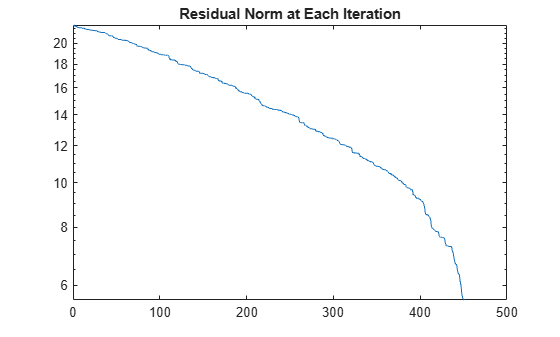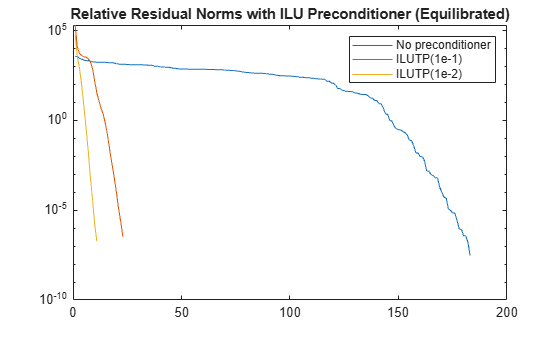# equilibrate

Matrix scaling for improved conditioning

## Syntax

``[P,R,C] = equilibrate(A)``

## Description

example

````[P,R,C] = equilibrate(A)` permutes and rescales matrix `A` such that the new matrix ```B = R*P*A*C``` has a diagonal with entries of magnitude 1, and its off-diagonal entries are not greater than 1 in magnitude.```

## Examples

collapse all

Equilibrate a matrix with a large condition number to improve the efficiency and stability of a linear system solution with the iterative solver `gmres`.

Load the `west0479` matrix, which is a real-valued 479-by-479 sparse matrix. Use `condest` to calculate the estimated condition number of the matrix.

```load west0479 A = west0479; c1 = condest(A)```
```c1 = 1.4244e+12 ```

Try to solve the linear system $\mathrm{Ax}=\mathit{b}$ using `gmres` with 450 iterations and a tolerance of `1e-11`. Specify five outputs so that `gmres` returns the residual norms of the solution at each iteration (using `~` to suppress unneeded outputs). Plot the residual norms in a semilog plot. The plot shows that `gmres` is not able to achieve a reasonable residual norm, and so the calculated solution for $\mathit{x}$ is not reliable.

```b = ones(size(A,1),1); tol = 1e-11; maxit = 450; [x,flx,~,~,rvx] = gmres(A,b,[],tol,maxit); semilogy(rvx) title('Residual Norm at Each Iteration')```Use `equilibrate` to permute and rescale `A`. Create a new matrix `B = R*P*A*C`, which has a better condition number and diagonal entries of only 1 and -1.

```[P,R,C] = equilibrate(A); B = R*P*A*C; c2 = condest(B)```
```c2 = 5.1036e+04 ```

Using the outputs returned by `equilibrate`, you can reformulate the problem $\mathrm{Ax}=\mathit{b}$ into $\mathrm{By}=\mathit{d}$, where $\mathit{B}=\mathrm{RPAC}$ and $\mathit{d}=\mathrm{RPb}$. In this form you can recover the solution to the original system with $\mathit{x}=\mathrm{Cy}$.

Use `gmres` to solve $\mathrm{By}=\mathit{d}$ for $\mathit{y}$, and then replot the residual norms at each iteration. The plot shows that equilibrating the matrix improves the stability of the problem, with `gmres` converging to the desired tolerance of `1e-11` in fewer than 200 iterations.

```d = R*P*b; [y,fly,~,~,rvy] = gmres(B,d,[],tol,maxit); hold on semilogy(rvy) legend('Original', 'Equilibrated', 'Location', 'southeast') title('Relative Residual Norms (No Preconditioner)') hold off```Improve Solution with Preconditioner

After you obtain the matrix `B`, you can improve the stability of the problem even further by calculating a preconditioner for use with `gmres`. The numerical properties of `B` are better than those of the original matrix `A`, so you should use the equilibrated matrix to calculate the preconditioner.

Calculate two different preconditioners with `ilu`, and use these as inputs to `gmres` to solve the problem again. Plot the residual norms at each iteration on the same plot as the equilibrated norms for comparison. The plot shows that calculating preconditioners with the equilibrated matrix greatly increases the stability of the problem, with `gmres` achieving the desired tolerance in fewer than 30 iterations.

```semilogy(rvy) hold on [L1,U1] = ilu(B,struct('type','ilutp','droptol',1e-1,'thresh',0)); [yp1,flyp1,~,~,rvyp1] = gmres(B,d,[],tol,maxit,L1,U1); semilogy(rvyp1) [L2,U2] = ilu(B,struct('type','ilutp','droptol',1e-2,'thresh',0)); [yp2,flyp2,~,~,rvyp2] = gmres(B,d,[],tol,maxit,L2,U2); semilogy(rvyp2) legend('No preconditioner', 'ILUTP(1e-1)', 'ILUTP(1e-2)') title('Relative Residual Norms with ILU Preconditioner (Equilibrated)') hold off```## Input Arguments

collapse all

Input matrix, specified as a square matrix. `A` can be dense or sparse, but must be structurally nonsingular, as determined by `sprank`.

When `A` is sparse, the outputs `P`, `R`, and `C` are also sparse.

Data Types: `single` | `double`
Complex Number Support: Yes

## Output Arguments

collapse all

Permutation matrix, returned as a matrix. `P*A` is the permutation of `A` that maximizes the absolute value of the product of its diagonal elements.

Row scaling, returned as a diagonal matrix. The diagonal entries in `R` and `C` are real and positive.

Column scaling, returned as a diagonal matrix. The diagonal entries in `R` and `C` are real and positive.

 Duff, I. S., and J. Koster. “On Algorithms For Permuting Large Entries to the Diagonal of a Sparse Matrix.” SIAM Journal on Matrix Analysis and Applications 22, no. 4 (January 2001): 973–96.